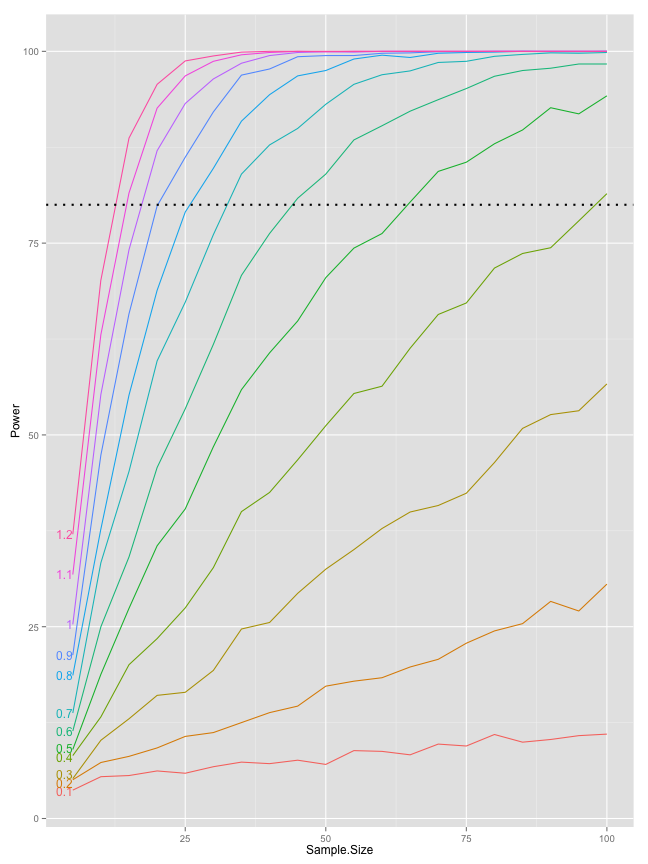## SET AND REP SCHEMES IN STRENGTH TRAINING (PART 2)

Here is a second installment of set and rep schemes article for EliteFTS. You can find my blog post on the first installment HERE

The purpose of the article is to 'explain' (or at least rise awareness) to the difference between Training objectives, Training parameters and Training progressions and variations. In simple words, training objectives represent description of what needs/can/should to be done to get from point A (current state) to point B (future state), defined by Needs Analysis and  Athlete Characteristic taking into account context at hand.

Training parameters then represent operational decisions and program in achieving training objectives.

Training progressions and variations represent a 'wiggle room' within Training parameters ~ since we can achieve same objectives using different approaches. The important point of the article is that there are similar ways to vary and progress training parameters regardless of training objectives. Those commonalities is what the article is set to explore.

In lay terms, if the training objective is to increase upper body muscle mass, we "know" (from research, previous experience or training knowledge) what training parameters generally needs to be performed  (e.g. training upper body 2-3x/wk with 30-50reps per muscle group with 65-80% 1RM), but within those parameters (and constraints) we have a lot of wiggle room to experiment with (art of coaching?). Here comes training progressions and variations that could be explored based on context, individual characteristics, reactions and preferences (e.g. sets across for someone, or waves for someone that hates sets across).

This is pretty much the same as Tool of Three Levels, just explained a bit differently.The explored progressions and variations are based on Load/Exertion profile (DOWNLOAD). Also, the new Strength Training Card Builder v3.0 will have ~90 set and reps schemes that are dependable on modifiable Load/Exertion table. If there is an interest will explain how I used Load/Exertion table to devise a lot of set and rep schemes.

Anyway, follow the links on the top of the page and let me know what you think.

## Thursday, February 13, 2014

### Continuing with Statitical Power simulation in R

Continuing with Statitical Power simulation in R

# Continuing with Statitical Power simulation in R

In the last blog post I created a simple simulation of statistical power (probability to identify effects when they are really there) calulation depending on the sample size and effect size (Cohen's D using Will Hopkins effect levels).

Now I will continue with the simulations and create 10 ES (from 0xSD [Baseline group] to 1xSD) levels with sample sizes going from 5 to 100 in 5 increment. We are going to resample 2000x

``````effect.magnitudes <- seq(from = 0, to = 1.2, length.out = 13)
subjects.list <- seq(from = 5, to = 100, by = 5)

p.value <- matrix(0, nrow = length(subjects.list), ncol = length(effect.magnitudes) -
1)

alpha <- 0.05

re.sampling <- 2000
significant.effects <- matrix(0, nrow = length(subjects.list), ncol = length(effect.magnitudes) -
1)

standard.deviation <- 30
sample.mean <- 100

for (k in 1:re.sampling) {
for (j in seq_along(subjects.list)) {
subjects <- subjects.list[j]
dataSamples <- matrix(0, nrow = subjects, ncol = length(effect.magnitudes))

for (i in seq_along(effect.magnitudes)) dataSamples[, i] <- rnorm(n = subjects,
mean = sample.mean + standard.deviation * effect.magnitudes[i],
sd = standard.deviation)

for (g in 2:(length(effect.magnitudes))) p.value[j, g - 1] <- t.test(dataSamples[,
1], dataSamples[, g])\$p.value
}

significant.effects <- significant.effects + (p.value < alpha)
}

significant.effects <- significant.effects/re.sampling * 100

significant.effects <- cbind(subjects.list, significant.effects)
colnames(significant.effects) <- c("Sample.Size", as.character(round(effect.magnitudes[-1],
2)))

significant.effects <- as.data.frame(significant.effects)
``````

We are going to plot the scores using ggplot2 and use direct labels

``````library(reshape2)
library(ggplot2)
library(directlabels)
``````
``````## Loading required package: grid
``````
``````
significant.effects.long <- melt(significant.effects, id.var = "Sample.Size",
value.name = "Power", variable.name = "Effect.Size")

gg <- ggplot(significant.effects.long, aes(x = Sample.Size, y = Power, color = Effect.Size))
gg <- gg + geom_line()
gg <- gg + geom_hline(yintercept = 80, linetype = "dotted", size = 1)
direct.label(gg, "first.qp")
``````
``````## Loading required package: proto
``````Using this graph one can estimate sample size for a wanted statistical power (usualy 80%) taking into account guessed effect size (by guessed I refer to an ES from pilot study, other research, etc). Once I get into multiple non-linear regression I can post the coefficients and create an equation. Till that happens, stay tuned :)